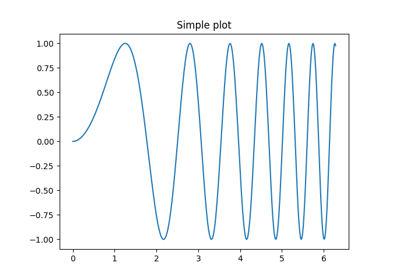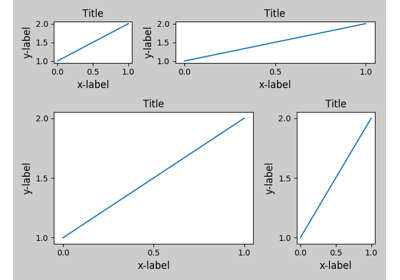matplotlib.pyplot.close¶

matplotlib.pyplot.close(*args)[source]

Close a figure window.

close() by itself closes the current figure

close(fig) closes the Figure instance fig

close(num) closes the figure number num

close(name) where name is a string, closes figure with that label

close('all') closes all the figure windows

Examples using matplotlib.pyplot.close¶Subplots DemoPipongMultipage PDFMultiprocessTight Layout guide